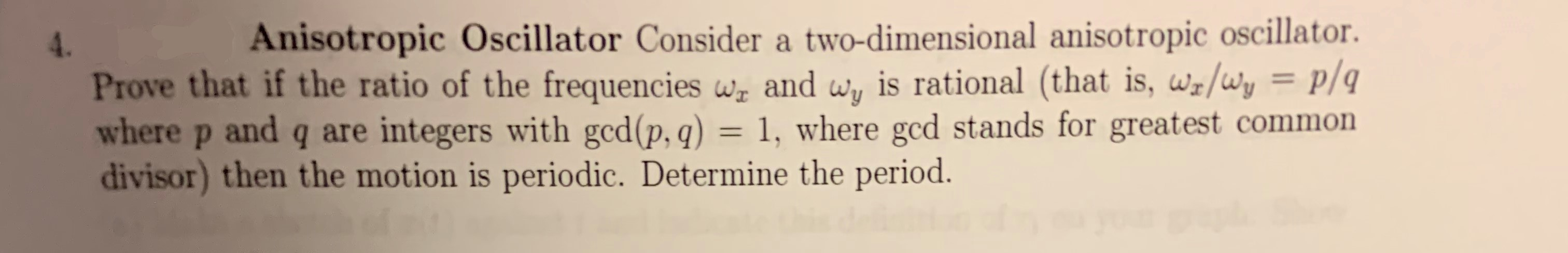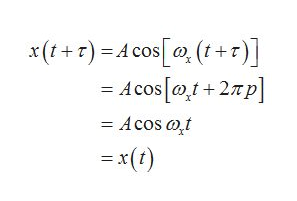Anisotropic Oscillator Consider a two-dimensional anisotropic oscillatoris rational (that is, ws/wy = p/q4.Prove that if the ratio of the frequencies w and wywhere p and q are integers with gcd(p, q) = 1, where gcd stands for greatest commondivisor) then the motion is periodic. Determine the period.P

Questionhelp_outlineImage TranscriptioncloseAnisotropic Oscillator Consider a two-dimensional anisotropic oscillator is rational (that is, ws/wy = p/q 4. Prove that if the ratio of the frequencies w and wy where p and q are integers with gcd(p, q) = 1, where gcd stands for greatest common divisor) then the motion is periodic. Determine the period. P fullscreen
Step 1

Assuming the ratio of the frequencies to be rational as mentioned in the problem.

Let τ be the smallest time period after which the motion along the x-direction is repeated.

This is expressed as,

Step 2

Here p is an integer, ωx is the frequency of oscillation of the anisotropic oscillator along the x axis.

From the relation between ωx and ωy given in the problem, τ can also be represented in terms of the frequency ωy of the anisotropic oscillator along y axis

Step 3

The solution for the anisotropic oscillator along ...help_outlineImage Transcriptionclosex(+r) Acos(t+r -Acos0+2p - Acos at = x(t) fullscreen

Want to see the full answer?

See Solution

Want to see this answer and more?

Our solutions are written by experts, many with advanced degrees, and available 24/7

See Solution
Tagged in

Science